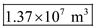# (a) The largest building in the world by volume is the Boeing 747 plant in Everett, Washington

(a) The largest building in the world by volume is the Boeing 747 plant in Everett, Washington. Tt measures approximately 631 m long, 707 yards wide, and 110 ft high. What is its volume in cubic feet? (b) Convert your result from part (a) to cubic meters.

Use the equation of volume of an object to calculate the volume of a building in cubit feet and cubic meter.
The equation for volume of a building is,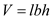Here, (l) is length ,(b) is width, and (h) is height.

Convert the units of length of the building from m to ft.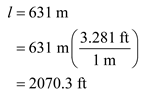Convert the units of length of the building from m to ft.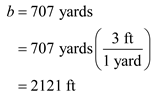(a)
The volume of the building in cubit feet is calculated as follows.
Substitute 2070.3 ft for(l),2121 ft for(b), and 110 ft for(h) in the equation of V = lbh and solve for volume of the building in cubit feet.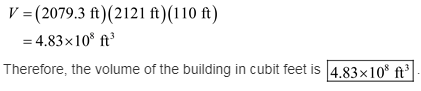(b)
The volume of the building in cubit feet is converted into cubic meters as follows.
Convert the units of length of the building from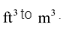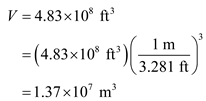Therefore, the volume of the building in cubic meters is